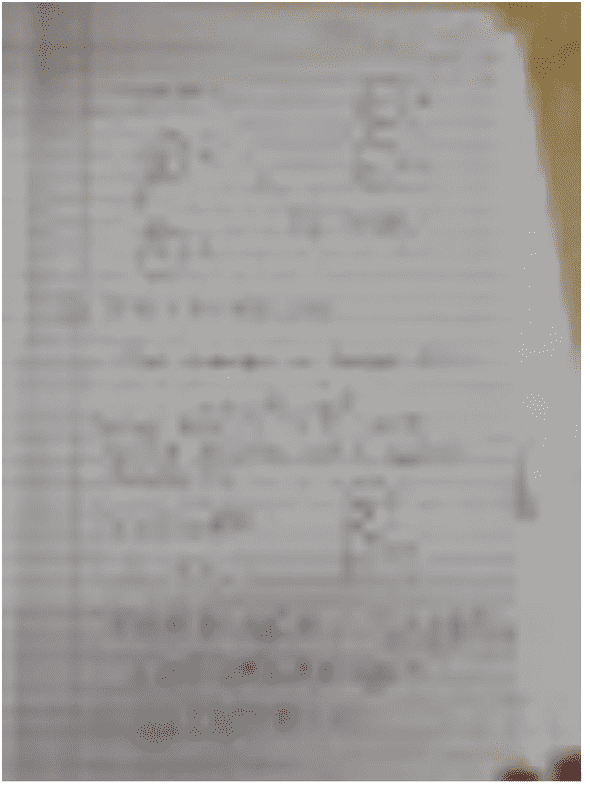# Time period of 2 disks connected by a spring

Vivek33010
Homework Statement:
2 identical disks (1 & 2) each of mass M and radius R are lying on a flat surface. They are free to rotate about their axis. They are connected by a spring of spring constant 'K'.
Find time period of small oscillations such that :-
Case A: spring 1 and 2 always rotate in same sense.
Case B: spring 1 and 2 always rotate in opposite sense.
Relevant Equations:
torque(τ) = (moment of inertia I)(angular acceleration α)
Equation of angular SHM, α = -(ω^(2))(θ)
My attempt at solving case B

I've attached my attempt at case B above. What problem I'm facing is that after writing equation of angular SHM, I'm getting angular acceleration proportional to cube of angular displacement, which doesn't reduce to SHM. So how to find time period for this oscillations, or how to reduce it to SHM?Proper diagram with question

Mentor
Homework Statement:: 2 identical disks (1 & 2) each of mass M and radius R are lying on a flat surface. They are free to rotate about their axis. They are connected by a spring of spring constant 'K'.
Find time period of small oscillations such that :-
Case A: spring 1 and 2 always rotate in same sense.
Case B: spring 1 and 2 always rotate in opposite sense.
Relevant Equations:: torque(τ) = (radius vector)×(force vector)
torque(τ) = (moment of inertia I)(angular acceleration α)
Equation of angular SHM, α = -(ω^(2))(θ)

My attempt at solving case B

I've attached my attempt at case B above. What problem I'm facing is that after writing equation of angular SHM, I'm getting angular acceleration proportional to cube of angular displacement, which doesn't reduce to SHM. So how to find time period for this oscillations, or how to reduce it to SHM?Proper diagram with question
Welcome to PF.Unfortunately, the image is unreadable. Maybe instead, check out the "LaTeX Guide" link below, and type your work into the Edit window? That makes it a lot easier to read and reply to. Thanks.Vivek33010
Welcome to PF.Unfortunately, the image is unreadable. Maybe instead, check out the "LaTeX Guide" link below, and type your work into the Edit window? That makes it a lot easier to read and reply to. Thanks.View attachment 281834
I write a new question or just edit this one?

Mentor
You can just reply below with the update to this problem. Or do you mean what you should do if you have a new/different question? In that case please start a new thread. Basically it is one thread per question/problem.

Vivek33010
You can just reply below with the update to this problem. Or do you mean what you should do if you have a new/different question? In that case please start a new thread. Basically it is one thread per question/problem.
I'll make a new thread, with the same question, but with better representation. I'll see if I can use latex because I don't know what it is. New thread coming right up

•berkeman
Mentor
Then I'll close this thread for now. Let me know if you want it back open (send me a message by clicking on my avatar and "Start a Conversation").